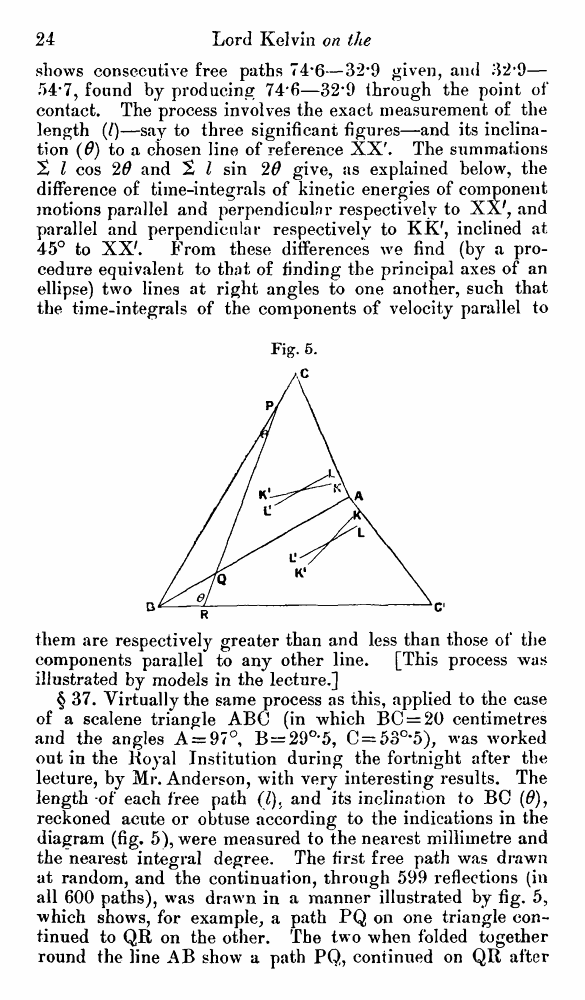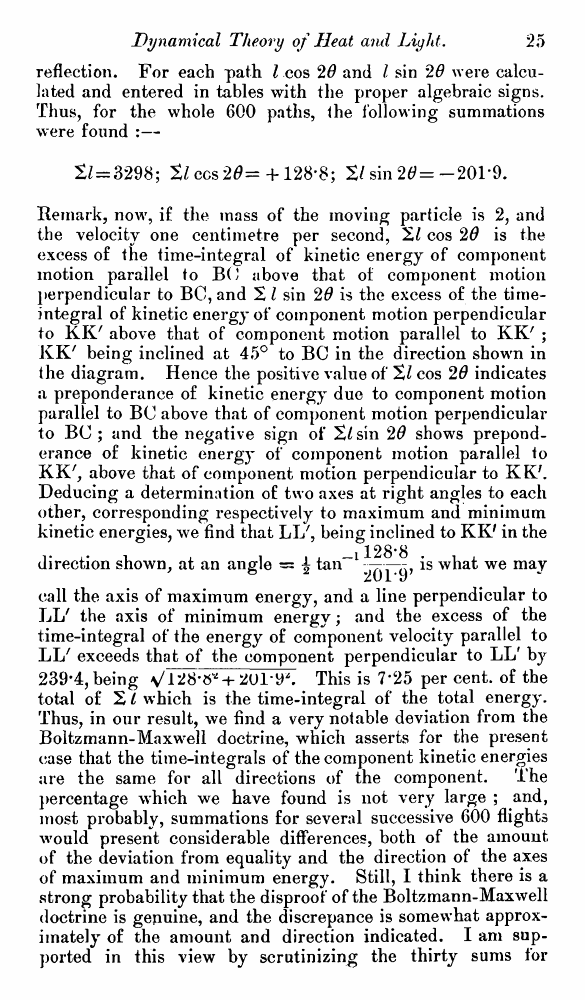# Lord Kelvin. Nineteenth-Century Clouds over the Dynamical Theory of Heat and Light. // Phil. Mag. S. 6. Vol. 2. No. 7. July 1901.

В начало   Другие форматы   <<<     Страница 24   >>>1  2  3  4  5  6  7  8  9  10  11  12  13  14  15  16  17  18  19  20  21  22  23  24 25  26  27  28  29  30  31  32  33  34  35  36  37  38  39  40 shows consecutive free paths 74*6—32*9 given, and 32*9— 54*7, found by producing 74*6—32*9 through the point of contact. The process involves the exact measurement of the length (/)—say to three significant figures—and its inclination (0) to a chosen line of reference XX'. The summations 2 I cos 20 and 2 I sin 20 give, as explained below, the difference of time-integrals of kinetic energies of component motions parallel and perpendicular respectively to XX', and parallel and perpendicular respectively to KK7, inclined at 45° to XX'. From these differences we find (by a procedure equivalent to that of finding the principal axes of an ellipse) two lines at right angles to one another, such that the time-integrals of the components of velocity parallel to Fig. 5. them are respectively greater than and less than those of the components parallel to any other line. [This process was illustrated by models in the lecture.] § 37. Virtually the same process as this, applied to the case of a scalene triangle ABC (in which BC = 20 centimetres and the angles A = 97°, B—29°'5, C = 53°‘5), was worked out in the Royal Institution during the fortnight after the lecture, by Mr. Anderson, with very interesting results. The length -of each free path (I). and its inclination to BC (0), reckoned acute or obtuse according to the indications in the diagram (fig, 5), were measured to the nearest millimetre and the nearest integral degree. The first free path was drawn at random, and the continuation, through 599 reflections (in all 600 paths), was drawn in a manner illustrated by fig. 5, which shows, for example, a path PQ on one triangle continued to QR on the other. The tw o when folded together round the line AB show a path PQ, continued on QR after reflection. For each path I cos 20 and I sin 20 were calculated and entered in tables with the proper algebraic signs. Thus, for the whole 600 paths, the following summations were found :— 2/ = 3298; tl ccs 20= + 128*8; 2/sin 20=-201'9. Remark, now, if the mass of the moving particle is 2, and the velocity one centimetre per second, 2/ cos 20 is the excess of the time-integral of kinetic energy of component motion parallel to BO above that of component motion perpendicular to BC, and 2 I sin 20 is the excess of the time-integral of kinetic energy of component motion perpendicular to KK7 above that of component motion parallel to KK' ; KK' being inclined at 45° to BC in the direction shown in the diagram. Hence the positive value of 21 cos 20 indicates a preponderance of kinetic energy due to component motion parallel to BC above that of component motion perpendicular to BC ; and the negative sign of 2/sin 20 shows preponderance of kinetic energy of component motion parallel to KK', above that of component motion perpendicular to KK/. Deducing a determination of two axes at right angles to each other, corresponding respectively to maximum and minimum kinetic energies, we find that LI/, being inclined to KK' in the 128*8 direction shown, at an angle = \ tan“l ^j—is what we may call the axis of maximum energy, and a line perpendicular to LL' the axis of minimum energy; and the excess of the time-integral of the energy of component velocity parallel to LL' exceeds that of the component perpendicular to LL' by 239-4, being s/1+ 2U1-9*. This is 7*25 per cent, of the total of 2/ which is the time-integral of the total energy. Thus, in our result, we find a very notable deviation from the Boltzmann-Maxwell doctrine, which asserts for the present case that the time-integrals of the component kinetic energies are the same for all directions of the component. The percentage which we have found is not very large ; and, most probably, summations for several successive 600 flights would present considerable differences, both of the amount of the deviation from equality and the direction of the axes of maximum and minimum energy. Still, I think there is a strong probability that the disproof of the Boltzmann-Maxwell doctrine is genuine, and the discrepance is somewhat approximately of the amount and direction indicated. I am supported in this view by scrutinizing the thirty sums for